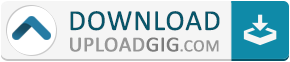#Do not remember me!

### Categories» » » Learn Python Quickly And C# Coding Practice Exercises: Coding For Beginners

## Learn Python Quickly And C# Coding Practice Exercises: Coding For BeginnersEnglish | July 2021 | ASIN : B098QRXNV7 | 127 pages | PDF, AZW3, EPUB | 1.72 MB

This book contains PYTHON BASICS AND JAVA coding exercises for beginners. It's useful Job interviews and college examinations. This book only for absolute beginners.

LEARN PYTHON QUICKLY
Python: Hello World program
Python interactive command line
Python: Operators
Arithmetic Operators in python
Relational Operators in python
Assignment operators in python
Logical Operators in python
Bitwise Operators in python
Membership operators in python
Identity operators in python
Python: Short circuit operators
Strings in python
Python: if condition
Python: while statement
Python: for statement
Python: break statement
Python: continue statement
Python: functions
Python: functions: return statement
Python lists
Python: tuples
Python: Class Vs Instance variables
Python: Inheritance
Python: Exceptions
Python: Handling Exceptions
Python global keyword
Python: Get type of variable
C# Sharp Basic Algorithm Exercises
Compute the sum
Get the absolute difference between n and 51
Check two given integers
Create a new string where 'if' is added
Remove the character in a given position of a given string
Exchange the first and last characters
Create a new string
New string with the last char
Check whether a given positive number is a multiple of 3
Check whether a given string starts with 'C#' or not
Check whether one of the given temperatures
Check two given integers
Check whether three given integer values
Check the largest number among three given integers
Print the output of multiplication
Print the average of four numbers
Program to convert Temperature
Compute the sum
Compute sum
C# program to check
C# Sharp program to check two given integers
C# Sharp program to check a given positive number
Calculate the perimeterName:* E-Mail: Security Code: *